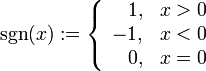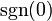# Signum function

(diff) ← Older revision | Latest revision (diff) | Newer revision → (diff)
The signum function, denoted$\operatorname{sgn}$, is defined as follows:$\operatorname{sgn}(x) := \left\lbrace \begin{array}{rl} 1, & x > 0 \\ -1, & x < 0 \\ 0, & x = 0 \\\end{array}\right.$
Note: In the definition given here, we define the value$\operatorname{sgn}(0)$ to be zero. In some alternative definitions,$\operatorname{sgn}(0)$ is considered to be undefined, i.e., the domain of$\operatorname{sgn}$ is taken to be the nonzero reals.